# Intro

Probabilistic Linear Discriminant Analysis (PLDA) is dimensionality reduction technique that could be seen as a advancement compared to Linear Discriminant Analysis (LDA). LDA features are derived as a result of training PLDA, but they have probability model attached to them, which automatically gives more weight to the more discriminative features (source).

Aim of this post is to show how PLDA is trained and used for inference. Finally few examples are showed how PLDA could be used for classification and clustering. I assume that you are familiar with concepts of LDA and have somewhat understanding of PLDA. If you are interested…

# Intro

Linear discriminant analysis (LDA) is a rather simple method for finding linear combination of features that distinctively characterize members in same classes and meantime separates different classes (source). This tutorial gives brief motivation for using LDA, shows steps how to calculate it and implements calculations in python Examples are available here.

# LDA

Briefly LDA tries to achieve two thing simultaneously:

• group samples from one class as close as possible (reduce in-class variance)
• separate samples from different classes as far as possible (increase between-class variance)

This property useful as it enables to cluster data, classify samples and/or reduce their dimensionality. Another popular…

# Baum-Welch algorithm

## Under the hood of Hidden Markov Models training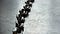Source

## Intro

This post aims to show how Hidden Markov Models (HMMs) are trained using Baum-Welch algorithm. If you want to learn more about Hidden Markov models, I suggest to read some posts:

This post assumes you are familiar with concepts like the transition, emission probabilities, hidden state, observation, forward and backward algorithm.

## Goal

Baum-Welch algorithm finds values for HMM parameters that best fit the observed data. For training we need:

• sequence of observations O₁, O₂, …, Oₙ
• sequence of hidden states for these observations S₁, S₂, …, Sₙ

Algorithm tries to…

# QR decomposition

## Calculating and using QR decomposition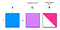Source

## Intro

QR decomposition (factorization) is decomposition of a matrix into orthogonal (Q) and upper triangular (R) matrices. QR factorization is used in solving linear least square problems and finding eigenvalues. This post shows how QR decomposition is computed and how to use it to solve practical problems.

## Gram-Schmidt Process

QR decomposition has following formula:

A = QR, where:

• A is original matrix we want to decompose
• Q is orthogonal matrix
• R is upper triangular matrix

Main goal is rather simple, decompose matrix into matrices Q and R. To find a orthogonal matrix Q, we could used Gram-Schmidt process. This process takes input matrix…

# LU decomposition

## Simple matrix decomposition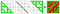Source

## Intro

LU (lower–upper) decomposition (factorization) outputs (factors original matrix into) lower and upper triangular matrix. These matrices could be used to efficiently solve system of non-sparse linear systems or find inverse of a matrix. It might sound a bit difficult, but we’ll have an example later. Goal of this post is to show what LU decomposition is about, how it could be calculated and used solving practical tasks.

## Gaussian elimination

In LU decomposition we want to decompose original into upper and lower triangular matrices, so that:

A = LU, where:

• A is original matrix we want to decompose
• L is lower triangular matrix…

# Simple SVD algorithms

## Naive ways to calculate SVD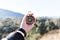Source

# Intro

Aim of this post is to show some simple and educational examples how to calculate singular value decomposition using simple methods. If you are interested in industry strength implementations, you might find this useful.

# SVD

Singular value decomposition (SVD) is a matrix factorization method that generalizes the eigendecomposition of a square matrix (n x n) to any matrix (n x m) (source).

If you don’t know what is eigendecomposition or eigenvectors/eigenvalues, you should google it or read this post. This post assumes that you are familiar with these concepts.

SVD is similar to Principal Component Analysis (PCA), but more general. PCA…

# Eigenvalues and Eigenvectors

## Explained by change of basis and transformation matrices.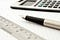Source

Eigenvalues have important role in data science. I’ll try to have my try explaining them. Before let’s dive into few topics that are crucial for understanding eigendecomposition. I assume that you are familiar with terms dot product, inverse of a matrix, orthogonal matrix.

Special thanks to Hadrien Jean and his book Essential Math for Data Science which was inspiration and main source of this article.

Code shown in this article is here.

## Change of basis

Change of basis means that we go from one basis system to another. Standard basis system (in two dimensions) graphs vector `[2, 1]` in the following way:

# Callbacks in neural networks

## Injecting changes into your models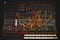Source

## Intro

While training machine learning model you want to play with it. Use different parameters, architectures, tricks and everything else that might improve performance. How to implement those changes? You have to change source code. But that will make codebase bigger, more difficult to maintain, more error-prone. Is there a better way? Yes! We could use callbacks.

Wikipedia defines callback as a “… any executable code that is passed as an argument to other code”. To my understanding, the main idea of callbacks is to inject code into existing program (functions, classes). …

# Fast.ai Practical Data Ethics series

## Notes based on course lessons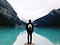Source

“This class was originally taught in-person at the University of San Francisco Data Institute in January-February 2020, for a diverse mix of working professionals from a range of backgrounds (as an evening certificate courses). There are no prerequisites for the course. This course is in no way intended to be exhaustive, but hopefully will provide useful context about how data misuse is impacting society, as well as practice in critical thinking skills and questions to ask.” (source)

Course syllabus could be found here.

This is list of lessons and my notes:

• Lesson 1: Disinformation. Video, notes
• Lesson 2: Bias &…

# Fast.ai Practical Data Ethics lesson 6 notes-Algorithmic Colonialism, and Next Steps

## How tech companies develop modern colonialism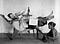Source

Lesson 6 materials are here. These are my notes and thus not complete and may contain mistakes.

# Algorithmic colonialism

Tech companies are sometimes developing colonialism. One example is Facebook’s Free Basics (source). It was going to help get people online and provide free basic access to internet. Indian government was against it, which in turn provoked Marc Andreessen to tweet something that he later regretted (and removed). But it clearly shows big tech companies attitude towards people in outside US.## Risto Hinno

Highly trained monkey